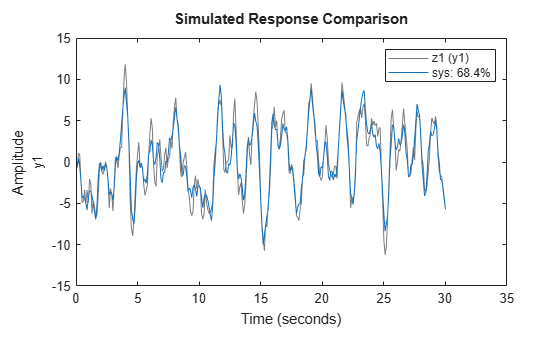# rpem

Estimate general input-output models using recursive prediction-error minimization method

## Syntax

```thm = rpem(z,nn,adm,adg)
```

## Description

`rpem` is not compatible with MATLAB® Coder™ or MATLAB Compiler™. For the special cases of ARX, AR, ARMA, ARMAX, Box-Jenkins, and Output-Error models, use `recursiveARX`, `recursiveAR`, `recursiveARMA`, `recursiveARMAX`, `recursiveBJ`, and `recursiveOE`, respectively.

The parameters of the general linear model structure

`$A\left(q\right)y\left(t\right)=\frac{{B}_{1}\left(q\right)}{{F}_{1}\left(q\right)}{u}_{1}\left(t-n{k}_{1}\right)+...+\frac{{B}_{nu}\left(q\right)}{{F}_{nu}\left(q\right)}{u}_{nu}\left(t-n{k}_{nu}\right)+\frac{C\left(q\right)}{D\left(q\right)}e\left(t\right)$`

are estimated using a recursive prediction error method.

The input-output data is contained in `z`, which is either an `iddata` object or a matrix ```z = [y u]``` where `y` and `u` are column vectors. (In the multiple-input case, `u` contains one column for each input.) `nn` is given as

```nn = [na nb nc nd nf nk] ```

where `na`, `nb`, `nc`, `nd`, and `nf` are the orders of the model, and `nk` is the delay. For multiple-input systems, `nb`, `nf`, and `nk` are row vectors giving the orders and delays of each input. See What Are Polynomial Models? for an exact definition of the orders.

The estimated parameters are returned in the matrix `thm`. The `k`th row of `thm` contains the parameters associated with time `k`; that is, they are based on the data in the rows up to and including row `k` in `z`. Each row of `thm` contains the estimated parameters in the following order.

```thm(k,:) = [a1,a2,...,ana,b1,...,bnb,... c1,...,cnc,d1,...,dnd,f1,...,fnf] ```

For multiple-input systems, the B part in the above expression is repeated for each input before the C part begins, and the F part is also repeated for each input. This is the same ordering as in `m.par`.

`yhat` is the predicted value of the output, according to the current model; that is, row `k` of `yhat` contains the predicted value of `y(k)` based on all past data.

The actual algorithm is selected with the two arguments `adg` and `adm`:

• `adm = 'ff'` and `adg` = `lam` specify the forgetting factor algorithm with the forgetting factor λ`=lam`. This algorithm is also known as recursive least squares (RLS). In this case, the matrix `P` has the following interpretation: R2`/2` * `P` is approximately equal to the covariance matrix of the estimated parameters.R2 is the variance of the innovations (the true prediction errors e(t)).

`adm ='ug'` and `adg = gam` specify the unnormalized gradient algorithm with gain gamma = `gam`. This algorithm is also known as the normalized least mean squares (LMS).

`adm ='ng'` and `adg` = `gam` specify the normalized gradient or normalized least mean squares (NLMS) algorithm. In these cases, `P` is not applicable.

`adm ='kf'` and `adg =R1` specify the Kalman filter based algorithm with R2=`1` and R1 = `R1`. If the variance of the innovations e(t) is not unity but R2; then R2* `P` is the covariance matrix of the parameter estimates, while R1 = `R1` /R2 is the covariance matrix of the parameter changes.

The input argument `th0` contains the initial value of the parameters, a row vector consistent with the rows of `thm`. The default value of `th0` is all zeros.

The arguments `P0` and `P` are the initial and final values, respectively, of the scaled covariance matrix of the parameters. The default value of `P0` is 104 times the unit matrix. The arguments `phi0`, `psi0`, `phi`, and `psi` contain initial and final values of the data vector and the gradient vector, respectively. The sizes of these depend on the chosen model orders. The normal choice of `phi0` and `psi0` is to use the outputs from a previous call to `rpem` with the same model orders. (This call could be a dummy call with default input arguments.) The default values of `phi0` and `psi0` are all zeros.

Note that the function requires that the delay `nk` be larger than `0`. If you want `nk = 0`, shift the input sequence appropriately and use `nk = 1`.

## Examples

### Estimate Model Parameters Using Recursive Prediction-Error Minimization

Specify the order and delays of a polynomial model structure.

```na = 2; nb = 1; nc = 1; nd = 1; nf = 0; nk = 1;```

`load iddata1 z1`

Estimate the parameters using forgetting factor algorithm with forgetting factor 0.99.

`EstimatedParameters = rpem(z1,[na nb nc nd nf nk],'ff',0.99);`

Get the last set of estimated parameters.

`p = EstimatedParameters(end,:);`

Construct a polynomial model with the estimated parameters.

```sys = idpoly([1 p(1:na)],... % A polynomial [zeros(1,nk) p(na+1:na+nb)],... % B polynomial [1 p(na+nb+1:na+nb+nc)],... % C polynomial [1 p(na+nb+nc+1:na+nb+nc+nd)]); % D polynomial sys.Ts = z1.Ts;```

Compare the estimated output with measured data.

`compare(z1,sys);`## Algorithms

The general recursive prediction error algorithm (11.44) of Ljung (1999) is implemented. See also Recursive Algorithms for Online Parameter Estimation.

### Topics

Introduced before R2006a

## SupportGet trial now